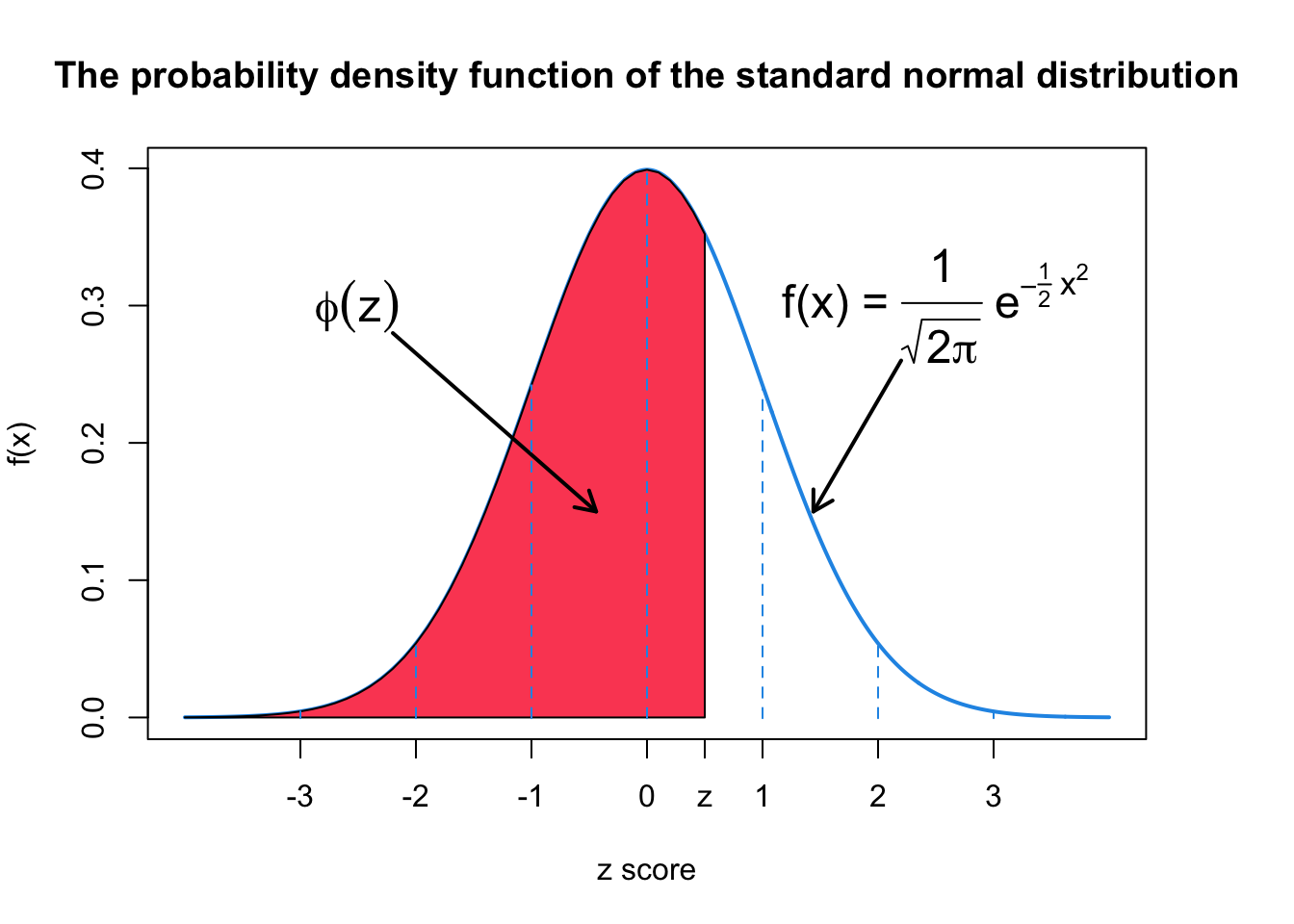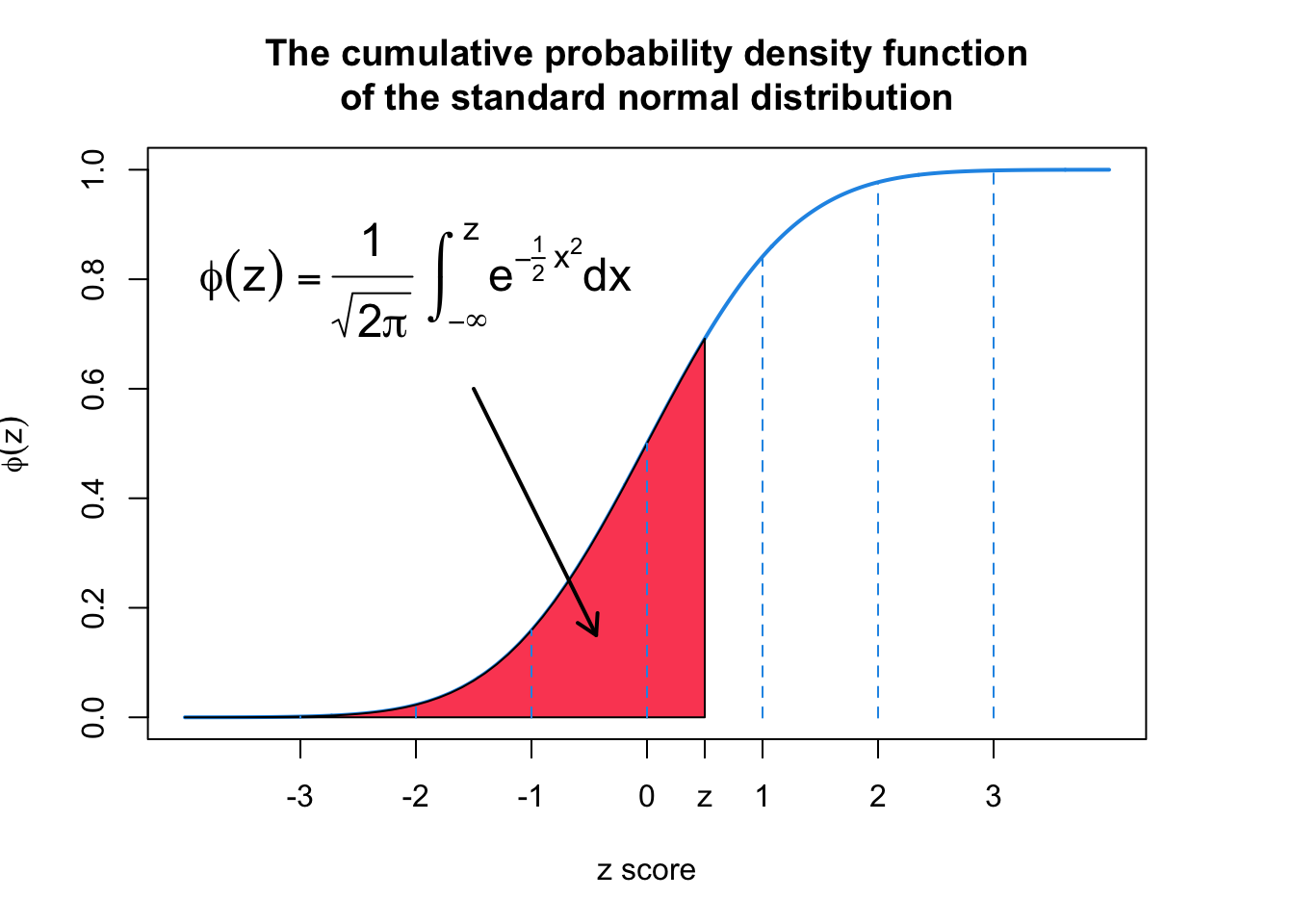The standard normal distribution is a special case of the normal distribution. For the standard normal distribution the value of the mean is equal to zero ($$\mu = 0$$) and the value of the standard deviation is equal to 1 ($$\sigma = 1$$).

Thus, by plugging in $$\mu = 0$$ and $$\sigma = 1$$ in the PDF of the normal distribution, the equation simplifies to:

\begin{align} f(x)& = \frac{1}{\sigma \sqrt{2 \pi}}e^{-\frac{1}{2}\left(\frac{x-\mu}{\sigma}\right)^2} \\ & =\frac{1}{1 \times \sqrt{2 \pi}}e^{-\frac{1}{2}\left(\frac{x-0}{1}\right)^2} \\ & = \frac{1}{\sqrt{2\pi}}e^{-\frac{1}{2}x^2} \end{align}

A random variable that follows the standard normal distribution is denoted by $$z$$. Consequently, the units for the standard normal distribution curve are denoted by $$z$$ and are called the $$z$$-values or $$z$$-scores. They are also called standard units or standard scores.

The cumulative distribution function (CDF) of the standard normal distribution, corresponding to the area under the curve for the interval $$(-\infty, z]$$, is usually denoted with the capital Greek letter $$\phi$$ and given by:

$F(x<z) = \phi (z) = \frac{1}{\sqrt{2\pi}} \int_{-\infty}^{z}e^{-\frac{1}{2}x^2}dx$

where $$e \approx 2.71828$$ and $$\pi \approx 3.14159$$.

#### Basic Properties of the Standard Normal Curve

The standard normal curve is a special case of the normal distribution and thus also a probability distribution curve. Therefore, basic properties of the normal distribution hold true for the standard normal curve as well (Weiss 2010).

1. The total area under the standard normal curve is 1 (this property is shared by all density curves).
2. The standard normal curve extends indefinitely in both directions, approaching, but never touching, the horizontal axis as it does so.
3. The standard normal curve is bell shaped and centered at $$z=0$$. Almost the whole area under the standard normal curve lies between $$z=-3$$ and $$z=3$$.The $$z$$-values on the right side of the mean are positive and those on the left side are negative. The $$z$$-value for a point on the horizontal axis gives the distance between the mean ($$z=0$$) and that point in terms of the standard deviation. For example, a point with a value of $$z=2$$ is two standard deviations to the right of the mean. Similarly, a point with a value of $$z=-2$$ is two standard deviations to the left of the mean.